• 所以有二项式展开: 很明显竖起来是等比，所以这样就可以把复杂度变成klogn了； 然后可以得出公式S结果公式为: 由于需要mod1e9+9，所以可以由费马小定理可知变形为: qi的值和阶乘的值都可以预处理，但是你会发现...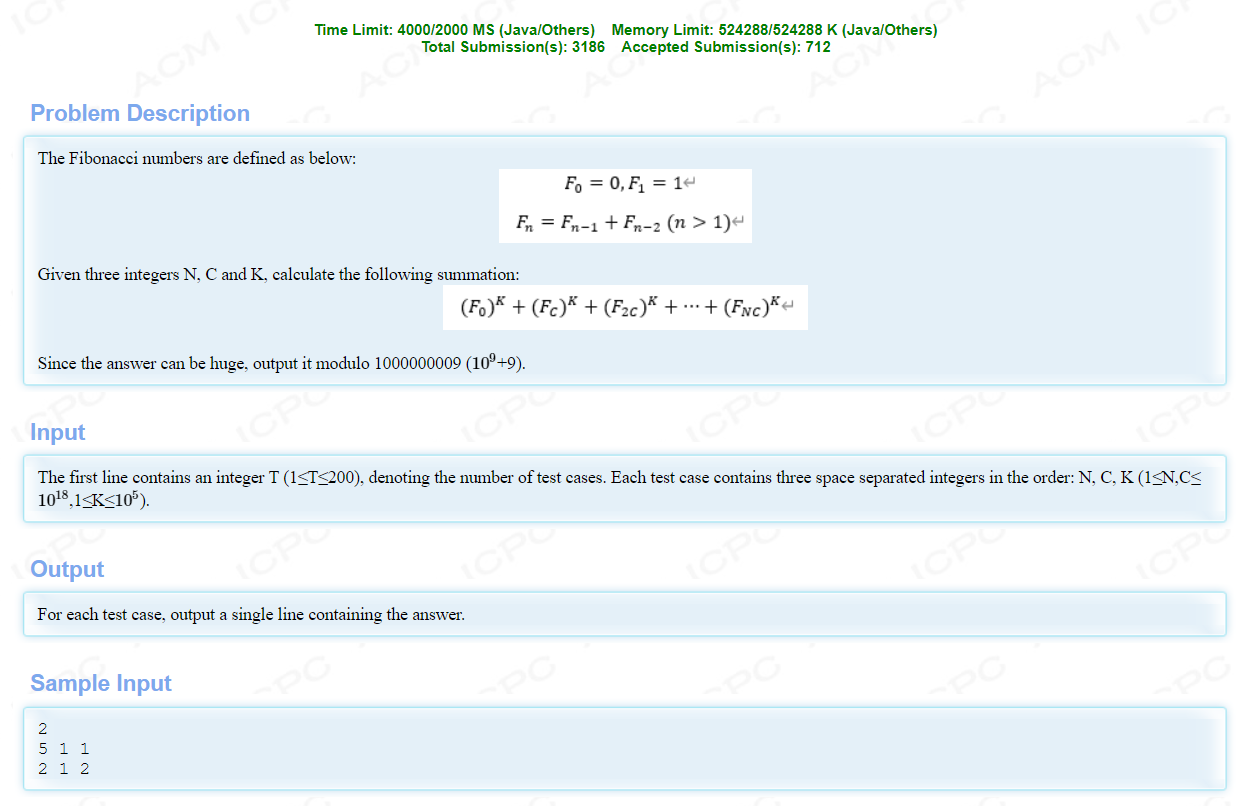这道题没写过类似的感觉确实不好想，考点在与二项式求和优化等式。特别是优化等式这里，是真的坑。
废话不多说，上推到过程:
因为: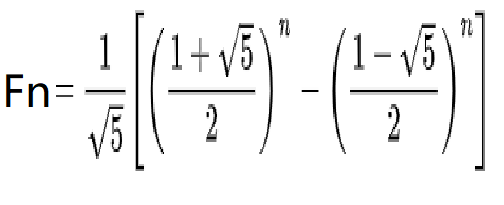可以有: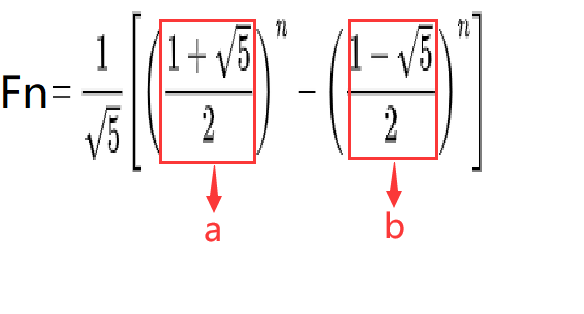所以有二项式展开: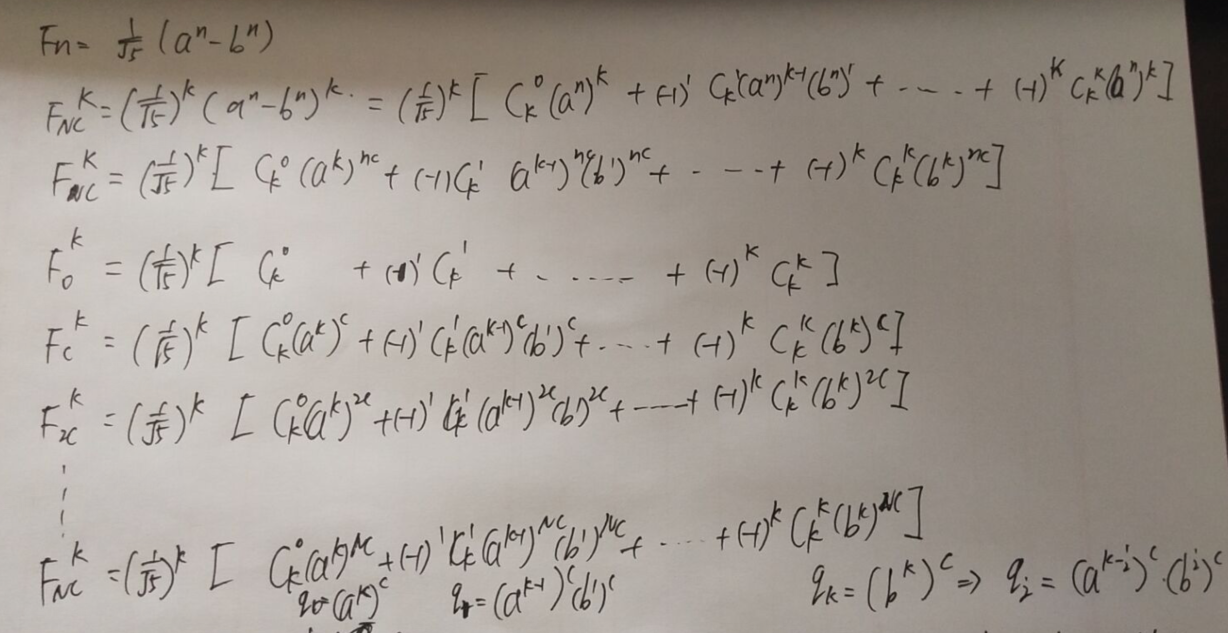很明显竖起来是等比，所以这样就可以把复杂度变成klogn了；
然后可以得出公式S结果公式为: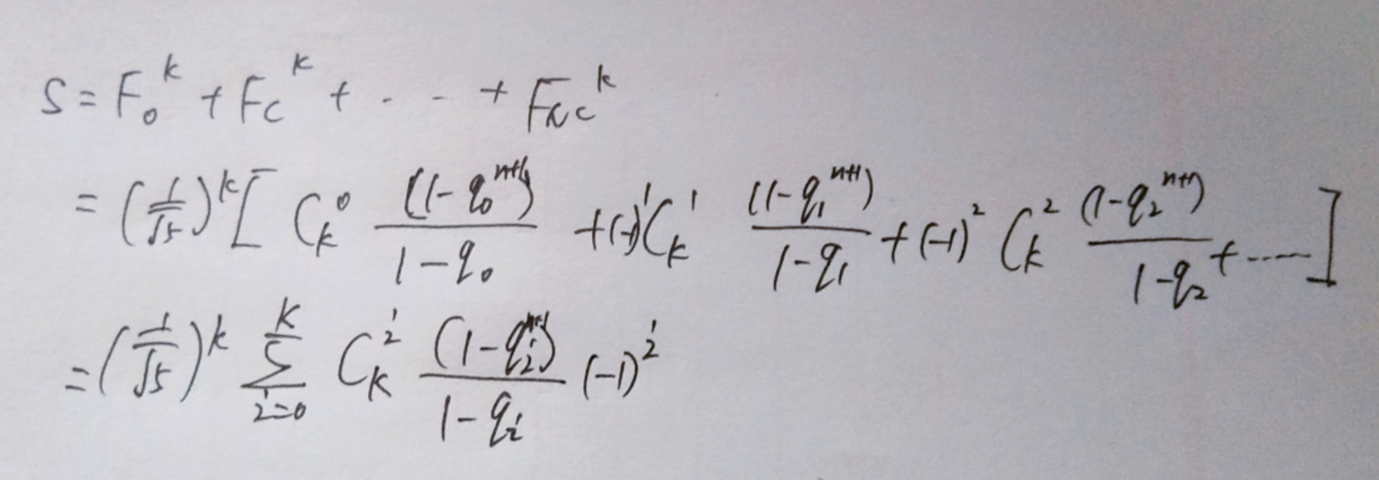由于需要mod1e9+9，所以可以由费马小定理可知变形为: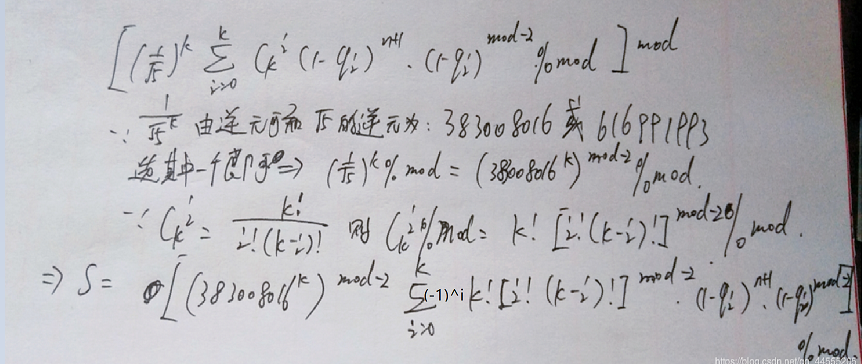qi的值和阶乘的值都可以预处理，但是你会发现如果直接带这个公式写出来就T了。这就是真的变态卡快速幂。。。。。。。。。
这是我超时的代码：
#include<bits/stdc++.h>
using namespace std;
typedef long long ll;
const ll P = 1e9+9;
const ll maxn = 1e5+5;
ll a[maxn],b[maxn],jie[maxn];
void pre(){
jie=1;
//预处理阶乘
for(ll i=1;i<maxn;i++)jie[i]=jie[i-1]*i%P;
//预处理a,b
a=1;b=1;
for(ll i=1;i<maxn;i++){
a[i]=a[i-1]*691504013%P;//a的%P的逆元
b[i]=b[i-1]*308495997%P;//b的%p的逆元
}
}
ll QP(ll x,ll n){
ll res=1;
while(n){
if(n&1){
res=res*x%P;
}
x=x*x%P;
n>>=1;
}
return res;
}
ll T;
ll N,C,K;
int main(){
scanf("%lld",&T);
pre();
ll ans=0;
ll x=QP(383008016,P-2);//利用费马小定理求1/sqrt(5)%P的值

while(T--){
ans=0;
scanf("%lld %lld %lld",&N,&C,&K);
ll t=QP(a[K],C)%P;
for(ll i=0;i<=K;i++){
ll fenzi=jie[K];
ll fenmu=jie[i]*jie[K-i]%P;
ll res=fenzi*QP(fenmu,P-2)%P;
ll temp=t*(QP(t,N)-1)%P*QP(t-1,P-2)%P;//这里有个十分奇葩的地方，我开头把他分开写居然wa了而不是T了。。。。。。。
if(t==1){//分母没有意义，求和可知为N
temp=N%P;
}
temp=temp*res%P;
if(i&1) ans-=temp;//如果为负数
else ans+=temp;//如果为正数
ans%=P;
}

ans=QP(x,K)*ans%P;
ans=(ans%P+P)%P;//防止负数
printf("%lld\n",ans);
}

return 0;
}


然后就很懵逼了，这怎么搞的？？？？？崩溃。。。。。。。
参考大佬的代码优化因为后一个q的值就是前一个q的值乘上A(-C)*B^C;
AC代码：
#include<bits/stdc++.h>
using namespace std;
typedef long long ll;
const ll mod=1e9+9;
const ll MAXN=1e5;
ll factor[MAXN+10];
ll invFactor[MAXN+10];
ll invn[MAXN+10];
const ll A=691504013;
const ll invA=691504012;
const ll B=308495997;
const ll D=276601605;
ll QP(ll x, ll n)
{
ll res=1;
while(n)
{
if (n&1) res=res*x%mod;
x=x*x%mod;
n>>=1;
}
return res;
}
ll Mod(ll a, ll b)
{
a+=b;
if (a>=mod)
a-=mod;
return a;
}
void pre()
{
factor=invFactor=invn=factor=invFactor=invn=1;
for(int i=2; i<=MAXN; i++)
{
factor[i]=factor[i-1]*i%mod;
invn[i]=(ll) (mod-mod/i)*invn[mod%i]%mod;
invFactor[i]=invFactor[i-1]*invn[i]%mod;
}
}
ll getC(ll m, ll n)//求C(n,m)
{
if (n<0 || m<0 || m>n)
return 0;
ll ans=factor[n];
ans=(ll) ans*invFactor[m]%mod;
ans=(ll) ans*invFactor[n-m]%mod;
return ans;
}
int main()
{
pre();
int T;
scanf("%d", &T);
while(T--)
{
ll n, c, k;
scanf("%lld%lld%lld", &n, &c, &k);
ll ans=0;
ll a1=QP(QP(A, k), c%(mod-1));
ll q=QP((ll) invA*B%mod, c%(mod-1));
ll n1=n%mod;
ll n2=n%(mod-1);
ll a1power=QP(a1, n2);
ll qpower=QP(q, n2);
for(int i=0; i<=k; i++)
{
ll sum=getC(i, k);
if (i&1)
{
sum=mod-sum;
}
if (a1==1)
{
ans=(ans+sum*n1%mod)%mod;
}
else
{
sum=sum*(a1*(a1power-1+mod)%mod)%mod;
sum=sum*QP(a1-1, mod-2)%mod;
ans=Mod(ans, sum);
}
a1=a1*q%mod;
a1power=a1power*qpower%mod;
}
printf("%lld\n",ans*QP(D,k)%mod);
}
}

实属想不到。。。。。。。


展开全文• 3、后面的就是二项式展开反着用往前推即可  例如最后一个式子那里sigma(2^k2*C(k3,k2))=(1+2)^k3 4、注意最后一次化简到的式子不能用二项式定理了，是一个等比数列求和 5、化成公式，费马小处理逆元，问题...

A Boring Question
Time Limit: 2000/1000 MS (Java/Others)    Memory Limit: 65536/65536 K (Java/Others)
Total Submission(s): 544    Accepted Submission(s): 313Problem Description
There are an equation.∑0≤k1,k2,⋯km≤n∏1⩽j<m(kj+1kj)%1000000007=?
We define that (kj+1kj)=kj+1!kj!(kj+1−kj)!
. And (kj+1kj)=0
while kj+1<kj.
You have to get the answer for each n
and m
that given to you.
For example,if n=1,m=3,
When k1=0,k2=0,k3=0,(k2k1)(k3k2)=1;
Whenk1=0,k2=1,k3=0,(k2k1)(k3k2)=0;
Whenk1=1,k2=0,k3=0,(k2k1)(k3k2)=0;
Whenk1=1,k2=1,k3=0,(k2k1)(k3k2)=0;
Whenk1=0,k2=0,k3=1,(k2k1)(k3k2)=1;
Whenk1=0,k2=1,k3=1,(k2k1)(k3k2)=1;
Whenk1=1,k2=0,k3=1,(k2k1)(k3k2)=0;
Whenk1=1,k2=1,k3=1,(k2k1)(k3k2)=1.

Input
The first line of the input contains the only integer T,(1≤T≤10000)
Then T
lines follow,the i-th line contains two integers n,m,(0≤n≤109,2≤m≤109)

Output
For each n
and m,output
the answer in a single line.

Sample Input

2
1 2
2 3

Sample Output

3
13

Author
UESTC

Source
2016 Multi-University Training Contest 6

Recommend
wange2014

题意……

解题思路
其实比赛的的时候哪有什么思路
本来想生推公式但是发现
除了找到非递降序列才有值这一有用规律就再不能看出什么了
也忘记了交换计算次序
从而变成二项式展开的形式
那就直接打表找规律呗……
ABORINGPROBLEM

正经化简如官方题解所示1、非递降序列有值
2、交换计算次序，把乘法对应的项提到自己做变元的那个累加号后
3、后面的就是二项式展开反着用往前推即可
例如最后一个式子那里sigma(2^k2*C(k3,k2))=(1+2)^k3
4、注意最后一次化简到的式子不能用二项式定理了，是一个等比数列求和
5、化成公式，费马小处理逆元，问题完美得解

#include <iostream>
#include <stdio.h>
#include <math.h>
using namespace std;

const int MO=1e9+7;
typedef long long LL;
LL qm(LL a,LL b)
{
LL ans=0;
while(b)
{
if(b&1)
ans=(ans+a)%MO;
a=(a+a)%MO;
b>>=1;
}
return ans;
}

LL qp(LL a,LL n)
{
LL ans=1;
while(n)
{
if(n&1)
ans=(ans*a)%MO;
a=(a*a)%MO;
n>>=1;
}
return ans;
}

int main()
{
int t;
int m,n;
scanf("%d",&t);
while(t--)
{
scanf("%d%d",&n,&m);
printf("%I64d\n",(qp(m,n+1)-1+MO)%MO*qp(m-1,MO-2)%MO);
}

return 0;
}


展开全文• 所以直接用二项式展开的系数表示组合数，对上指标隔项求和就可以表示为多项式的等比数列： 除 (x+1)2−1(x+1)^2-1(x+1)2−1，发现上下两项常数项为0，其实就是除 x−2x-2x−2。 可以发现有 ai=2bi+bi−1a_i=2b_i+b_...
题目描述：
给出$N,M,K$，求$\sum_{i=0}^N\sum_{j=0}^M\binom ij[i\!\!\!\!\mod2=0][j\!\!\!\!\mod2=0]$
$N\le10^9,M\le10^6,K\le10^9$
题目分析：
要是把组合数换成下降幂，斯特林数没法求，枚举复杂度也很高。。
所以直接用二项式展开的系数表示组合数，对上指标隔项求和就可以表示为多项式的等比数列：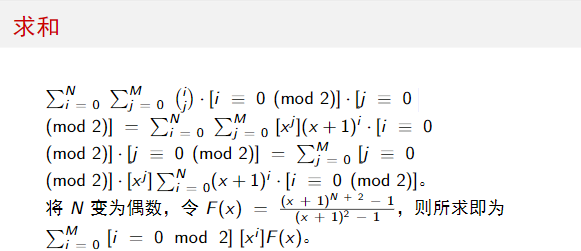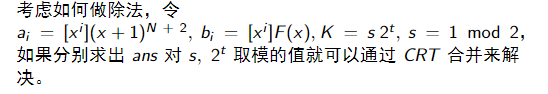除$(x+1)^2-1$，发现上下两项常数项为0，其实就是除$x-2$。
可以发现有$a_i=2b_i+b_{i-1}$，其中$a_i=\binom {N+2}{i+1}$

模数为奇数时，存在$2$的逆元，所以$b_0=a_0*2^{-1}$，$b_i=(a_i-b_{i-1})*2^{-1}(i>0)$：
模数为$2^t$时，不存在$2$的逆元，但是因为$b_i=\sum_{j>i}(-2)^{j-(i+1)}a_j$，当$j-(i+1)\ge t$时余数为0，所以可以直接算出$b_M=\sum_{t+M\ge j>i}a_j$，然后用$b_{i-1}=a_i-2b_i$递推。

所以最后的问题就是怎么算$a_i$，因为要对每一个$0\le i\le M$都算出$\binom {N+2}{i+1}$，所以首先需要将模数拆分为质数的幂，然后用类似$exlucas$的方法将下降幂和阶乘中的$p$的因子提出，剩下的数用$exgcd$求出逆元。于是可以$O(m\log m)$算出前$M$个组合数。
最后将所有拆分的模数$CRT$合并即可。
Code：
#include<bits/stdc++.h>
#define maxn 1000105
using namespace std;
int n,m,K,T,ans,mod,b,pw[maxn],C[maxn],inv[maxn],tmp;
void exgcd(int a,int b,int &x,int &y){
if(!b) {x=1,y=0;return;}
exgcd(b,a%b,y,x),y-=a/b*x;
}
void calc(const int p,const int k,int M){
int cnt=0,sum=1;
for(int i=pw=1;i<=k;i++) pw[i]=pw[i-1]*p; const int pk = pw[k];
for(int i=1,lim=min(M,pk-1);i<=lim;i++) if(i%p) exgcd(i,pk,inv[i],tmp);
for(int i=1;i<=M;i++){
int x=n+2-i+1,y=i;
if(x) for(;x%p==0;x/=p,cnt++);
for(;y%p==0;y/=p,cnt--);
sum=1ll*sum*x%pk*inv[y%pk]%pk;
C[i] = cnt>=k ? 0 : 1ll*sum*pw[cnt]%pk;
}
}
void merge(int R,int P){
int i1,i2;
exgcd(mod,P,i1,i2); const int md = mod*P;
ans=(1ll*ans*P%md*i2+1ll*R*mod%md*i1)%md, mod=md;
}
int main()
{
scanf("%d%d%d",&n,&m,&K),n-=(n&1),m-=(m&1),m=min(n,m);
ans=0,mod=1;

while(!(K&1)) K>>=1,T++;
if(T){
calc(2,T,min(m+1+T,n+2)); const int md = 1<<T;
for(int i=min(m+1+T,n+2);i>m+1;i--) b=((-2ll)*b+C[i])%md;
int R=b;
for(int i=m-1;i>=0;i--){
b=((-2ll)*b+C[i+2])%md;
if(!(i&1)) R=(R+b)%md;
}
merge(R,md);
}

for(int i=3,x;i*i<=K;i++) if(K%i==0){
for(x=0; K%i==0; K/=i,x++);
calc(i,x,m+1); const int md=pw[x],iv2=(md+1)/2;
int R=b=1ll*C*iv2%md;
for(int j=1;j<=m;j++){
b=1ll*(C[j+1]-b)*iv2%md;
if(!(j&1)) R=(R+b)%md;
}
merge(R,md);
}
if(K>1){
calc(K,1,m+1); const int md=K,iv2=(md+1)/2;
int R=b=1ll*C*iv2%md;
for(int j=1;j<=m;j++){
b=1ll*(C[j+1]-b)*iv2%md;
if(!(j&1)) R=(R+b)%md;
}
merge(R,md);
}
printf("%d\n",(ans+mod)%mod);
}



展开全文• ## 二项式定理

千次阅读 2018-08-14 11:07:10
二项式定理，又称牛顿二项式定理，此定理给出两个数之和的整数次幂诸如展开为类似项之和的恒等式。二项式定理可以推广到任意实数次幂，即广义二项式定理  可以将x+y的任意次幂展开成和的形式 其中每个   为...


二项式定理

二项式定理，又称牛顿二项式定理，此定理给出两个数之和的整数次幂诸如展开为类似项之和的恒等式。二项式定理可以推广到任意实数次幂，即广义二项式定理

可以将x+y的任意次幂展开成和的形式其中每个为一个称作二项式系数的特定正整数，其等于。这个公式也称二项式公式或二项恒等式。使用求和符号，可以把它写作展开全文• 这种东西显然需要直接上斐波那契通公式。 设x=1+52,y=1−52,z=15x=\frac{1+\sqrt 5}{2},y=\frac{1-\sqrt 5}{2},z=\frac{1}{\sqrt 5}x=21+5​​,y=21−5​​,z=5​1​，则我们要求的就是这个东西： Ans=∑i=1nzk⋅...
• 容易忘记的公式备用： arithmetic series geometric series permutation combination n number set has 2^n subsets n length string has n! permutation binomial 二项式string
• 一、组合恒等 ( 递推 ) 、 、组合恒等 ( 变下项求和 ) 简单和 、 、组合恒等 ( 变下项求和 ) 交错和 、
• 必胜，现在问题在于我们要求和啊，只知道单独局面的结果是无法求和的，我们需要一个游戏值，一个衡量赢面有多大的东西。 前面已经说了这个东西并不能用实数衡量， 也就是说不能求和 ，而我们实际要考虑的其实就是...
• ,那么以此二项式展开，除了第一项 C 0 n a b C n 0 a b C_n^0a^b ,其他项都会带有7这个项数，所以都会被7整除，得证。 所以我们就可以按照指数分类了 如： 1 + 1 8 + 1 15 + . . . + 1 7 k − 6 1 + 1 8 + 1 ...
• 二项式定理可以将x+y的任意次幂展开成和的形式 其中每个  为一个称作二项式系数的特定正整数，其等于 。 这个公式也称二项式公式或二项恒等式。使用求和符号，可以把它写作 很明显，当x==y==1时，会有下面这...
• (ax+b)n(ax+b)^n(ax+b)n的展开式中，第r+1项的二项式系数为 CnrC_n^rCnr​ 第r+1项的系数为 Cnran−rbrC_n^ra^{n-r}b^rCnr​an−rbr 而 (x+1x)n(x+\frac{1}{x})^n(x+x1​)n的展开式中的系数等于二项式系数 二项式...AI 数学 二项分布
• 先是从二项式平方开始: 其实展开是这样的: 再看立方: 通过排列组合的方式标记, 于是: 通过数学归纳法可以拓展: 使用求和简写可得: e 级数 数学常数 e (The Constant e – NDE/NDT Resource ...
• [CQOI2015]&[bzoj3933]多项式　二项式定理＋高精度数学 高精度算法
• 排列组合相关与二项式基础 一、加乘原理 1.加法原理 做一件事，完成他的方法可以分为$$n$$个互不相交的类，每一类有$$a_i$$中方法，则完成这件事一共有 $a_1+a_2+...+a_n$ 种方法。 加法原理的核心思想就是，把...
• 1.公式 首先我们都知道组合数的意义，就是说一共有n个样本，一次性从中取出m个样本，一共有多少种不同的取法。它的公式如下： 它有这么一个性质： ...首先知道，(a+b) ^n 的展开式一共有n+1...组合数性质
• 二项式系数，也是我们常用的组合数，最直观的组合意义就是从n个元素取k个元素所有可能的情况数，因此我们自然的得到下面二项式系数的定义式。 那么我们通过具有组合意义的二项系数，给出更加一般的二项式系数的...
• 二项式定理好难啊...学了好久 $$QWQ$$ 这篇博客写的有点杂，主要讲证明，仅供娱乐？ 二项式定理的常见形式 首先我们看看这个常见的令人头疼的式子： $(x+1)^n=\sum_{i=0}^{n} C(n,i) ~ x^i$ 这个式子为什么是对的...
• 1.题目链接 ...2.解题思路 首先，n在k进制下展开： n=k ^ 0 + k ^ 1 + k ^ 2 + …+ k ^ m > k ^ m; 展开式可以看作一个首项为1，公比为...根据二项式定理: (1+k) ^ m > n > k ^ m ; 那么有 k < pow(n,1/m) <
• 题目大意问有多少种方案...分析很容易发现，长度为ii合法01串个数为Fi+2F_{i+2}(FiF_i表示斐波那契数列的第i)，方案数就为(Fi+2k)F_{i+2}\choose k，令Sn=∑n+2i=3(Fik)S_n=\sum _{i=3}^{n+2} {F_{i}\choose k}，则c++ 斐波那契数列 第一类斯特林数 codeforces
• 二项式定理 帕斯卡公式和帕斯卡三角形 帕斯卡公式 对于 0 ⩽ r ⩽ n 0\leqslant r\leqslant n 0 ⩽ r ⩽ n ，有 ( n r ) = ( n n − r ) {n\choose r} ={n\choose n-r} ( r n ​ ) = ( n − r n ​ ) 证明 ...
• 数论(二次剩余 + 二项式定理 + 斐波那契数列) - Fibonacci Sum - HDU 6755 题意： 斐波那契数列：斐波那契数列：斐波那契数列： {F0=0,F1=1Fn=Fn−1+Fn−2(n>1)\begin{cases}F_0=0,F_1=1\\\\F_n=F_{n-1}+F_{n-2}...算法 斐波那契数列 费马小定理
• 文章目录生成函数引言定义有关幂级数的有用事实形式幂级数幂级数的和、积广义二项式定理常见的生成函数使用生成函数解决计数问题使用生成函数求解递推关系使用生成函数证明恒等式 生成函数 引言 定义 实数序列a0、a1......